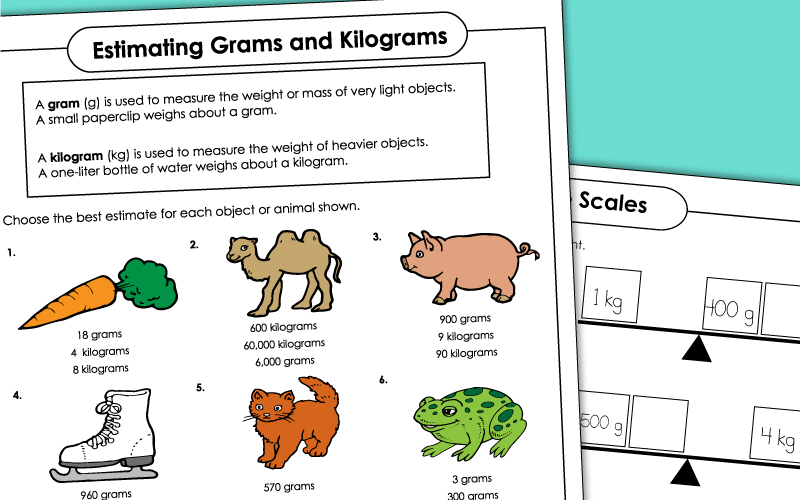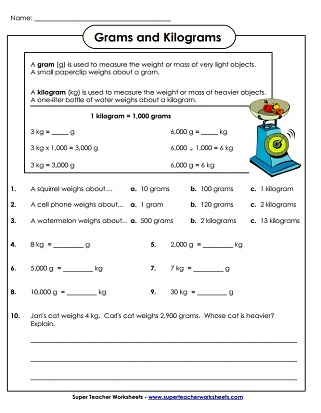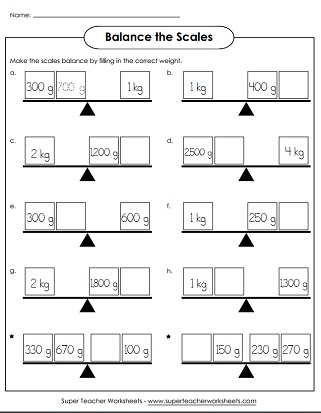# Measure Weight

These measurement worksheets will help students learn to: measure the mass or weight of objects in metric units (kilograms and grams), estimate the mass of given objects, convert mass from grams to kilograms and vice-versa.Circle the best mass estimate (grams and kilograms) for each of the objects pictured.
A slightly harder version of the worksheet above; Convert weight amounts to and from grams and  kilograms; Includes decimal numbers
A page of word problems for measuring weight in grams and kilograms.
Calculate the correct mass amounts to make the scales balance.

## Also on Super Teacher Worksheets...

Capacity (Gallons, Quarts, etc.)

Measure capacity/volume using cups, quarts, pints, and gallons

Linear Measurement (Centimeters, Millimeters)

Measure to the nearest millimeter or centimeter; Metric measurement printables

## Sample Worksheet ImagesMy Account
Site Information